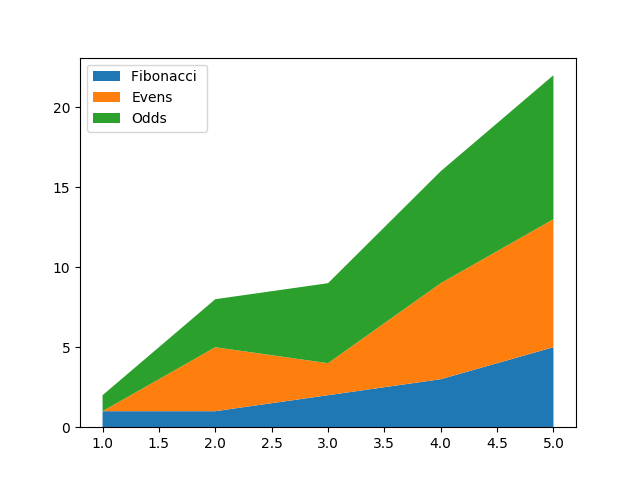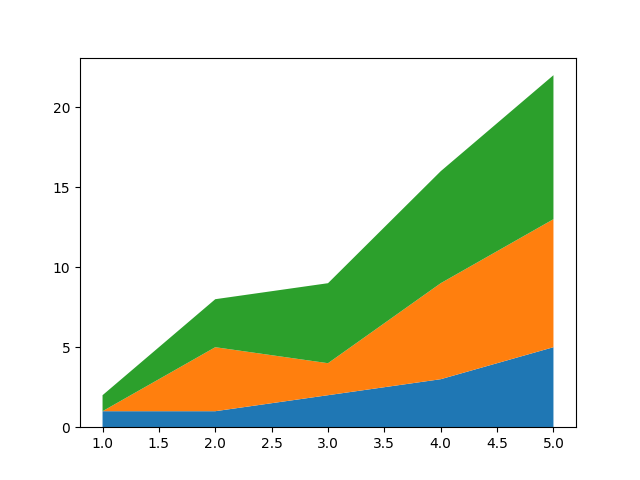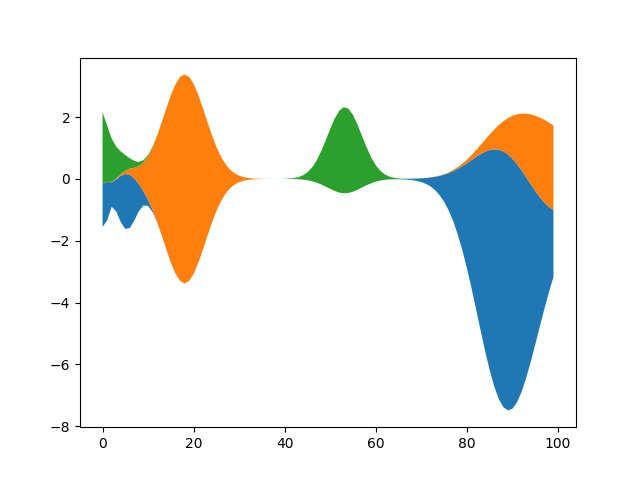Stackplot Demo¶

How to create stackplots with Matplotlib.

Stackplots are generated by plotting different datasets vertically on top of one another rather than overlapping with one another. Below we show some examples to accomplish this with Matplotlib.

import numpy as np
import matplotlib.pyplot as plt

x = [1, 2, 3, 4, 5]
y1 = [1, 1, 2, 3, 5]
y2 = [0, 4, 2, 6, 8]
y3 = [1, 3, 5, 7, 9]

y = np.vstack([y1, y2, y3])

labels = ["Fibonacci ", "Evens", "Odds"]

fig, ax = plt.subplots()
ax.stackplot(x, y1, y2, y3, labels=labels)
ax.legend(loc=2)
plt.show()

fig, ax = plt.subplots()
ax.stackplot(x, y)
plt.show()
••Here we show an example of making a streamgraph using stackplot

def layers(n, m):
"""
Return *n* random Gaussian mixtures, each of length *m*.
"""
def bump(a):
x = 1 / (.1 + np.random.random())
y = 2 * np.random.random() - .5
z = 10 / (.1 + np.random.random())
for i in range(m):
w = (i / m - y) * z
a[i] += x * np.exp(-w * w)
a = np.zeros((m, n))
for i in range(n):
for j in range(5):
bump(a[:, i])
return a

d = layers(3, 100)

fig, ax = plt.subplots()
ax.stackplot(range(100), d.T, baseline='wiggle')
plt.show()Keywords: matplotlib code example, codex, python plot, pyplot Gallery generated by Sphinx-Gallery